• 程序的基本语法元素
程序的格式框架、缩进、注释、变量、命令、保留字、数据类型、赋值语句、引用
• 基本输入、输出函数
input()、eval()、print()
• 源程序的书写风格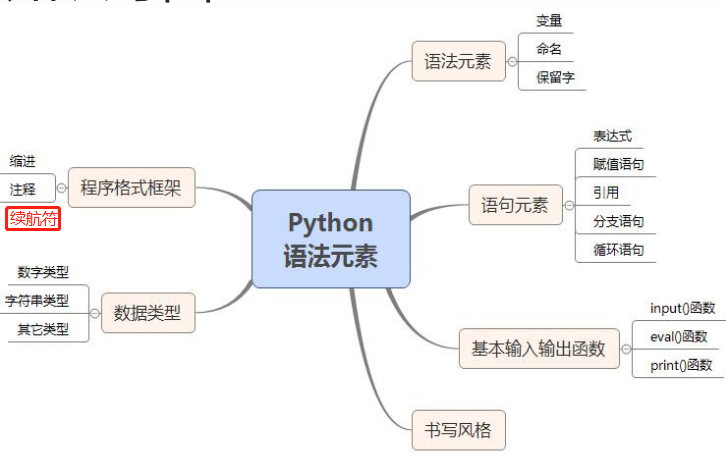# Python程序的格式框架

## 缩进

• 1个缩进 = 4个空格
• 缩进是Python语言中表明程序框架的唯一手段
• 一般代码不需要缩进，顶行编写且不留空白
• 当表达分支、循环、函数、类等程序含义时，
在if、while、for、def、class等保留字所在完整
语句后通过英文冒号（:）结尾并在之后进行缩
进，表明后续代码与紧邻无缩进语句的所属关

### 缩进错误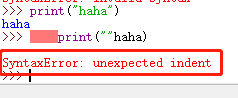## 注释

``````>>> #注释的第一行
#注释第二行
print("hahaha")#注释开始

hahaha
``````

## 续行符

• Python每行代码并无长度限制
• 提供续行符（由反斜杠\符号表示）将但行代码分割为多行表达式
``````>>> print("{}是{}的首都".format(\
"北京",\
"中国"\
))

>>> print("{}是{}的首都".format("北京","中国"))

``````

# 语法元素的名称

Python的少部分单词为保留字，大部分由用户自己定义，通过命名过程变成了变量或函数

## 变量

``````>>> a=1
>>> a=a+1
>>> a+=1
>>> print(a)
3
``````

## 命名

• 允许采用大写字母、小写字母、数字、下划线(_)和汉字等字符及其组合给变量命名，但名字的首字符不能是数字，中间不能出现空格，长度没有限制
注意：标识符对大小写敏感，python和Python是两个不同的名字

## 保留字

Python 3.5 有35个保留字，区分大小写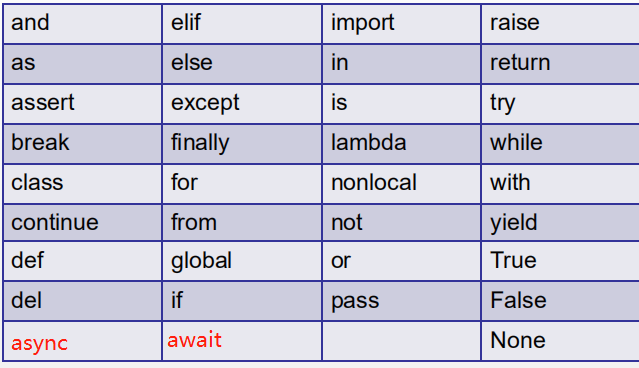``````>>> true==True
Traceback (most recent call last):
File "<pyshell#50>", line 1, in <module>
true==True
NameError: name 'true' is not defined
>>> True==True
True
>>>
``````

# 数据类型

Python语言支持多种数据类型，最简单的包括数字类型、字符串类型，略微复杂的包括元组类型、集合类型、列表类型、字典类型等

## 数字类型

Python语言提供3种数字类型：整数、浮点数和复数，分别对应数学中的整数、实数和复数

• 整数
一个整数值可以表示为十进制、十六进制、八进制和二进制等不同进制形式
``````>>> 1010==0x3F2
True
``````
• 浮点数
一个浮点数可以表示为带有小数点的一般形式，也可以采用科学计数法表示
• 复数
复数类型与数学中的复数相一致，采用a+bj的形式表示，存在实部和虚部。

## 字符串类型

### 序号体系

Python字符串的两种序号体系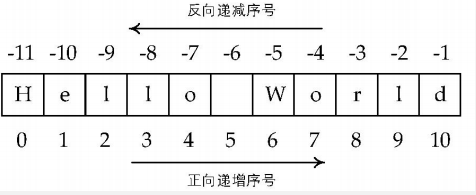• 截取单个字符
如果字符串长度为L，正向递增需要以最左侧字符序号为0，向右依次递增，最右侧字符序号为L-1；
反向递减序号以最右侧字符序号为-1，向左依次递减，最左侧字符序号为-L
``````>>> "对酒当歌,人生几何?"
'酒'
>>> "对酒当歌,人生几何?"[-1]
'?'
>>> "对酒当歌,人生几何?"
'歌'
>>> "对酒当歌,人生几何?"[-3]
'几'
``````
• 截取子串
可以采用[N: M]格式获取字符串的子串，这个操作被形象地称为切片。[N: M]获取字符串中从N到M（但不包含M，半闭半开集合）间连续的子字符串
"譬如朝露,去日苦多。"[2:4]
``````>>> '譬如朝露,去日苦多。'[2:4]
'朝露'
>>> "譬如朝露,去日苦多。"[2:4]
'朝露'
>>> "譬如朝露,去日苦多。"[2:2]
''
>>> "譬如朝露,去日苦多。"[2:1]
''
>>> "譬如朝露,去日苦多。"[1:2]
'如'
``````
• 获取字符串长度
Python默认提供的len()函数获取字符串的长度，一个中文字符和西文字符的长度都记为1
``````>>> len("譬如朝露,去日苦多。")
10
>>> a=("譬如朝露,去日苦多。")
>>> len(a)
10
``````

# 程序的语句元素

## 赋值语句

Python语言中，= 表示“赋值”

• 赋值单个
<变量> = <表达式>
• 同步赋值
1.同时给多个变量赋值
<变量1>, …, <变量N> = <表达式1>, …, <表达式N>
``````>>> n=3
>>> x,y=n+1,n+2
>>> x
4
>>> y
5
``````

2.相互交换变量的值

``````>>> x,y=y,x
>>> x
5
>>> y
4
``````

## 引用

Python程序会经常使用当前程序之外已有的功能代码，这个过程叫“引用”
。Python语言使用import保留字引用当前程序以外的功能库，使用方

import <功能库名称>

<功能库名称>.<函数名称>()方式调用具体功能，简称为A.B()

``````import turtle
turtle.fd(-200)
turtle.right(90)
turtle.circle(200)
``````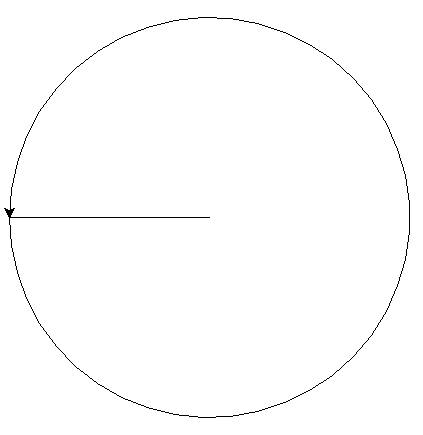## 其他语句

Python程序还包括一些其他的语句类型，例如，分支语句和循环语句等

### 分支语句

• 单分支
if <条件>:
<语句块>
``````num=eval(input("请输入一个整数："))
if 0<=num<=100:
print("输入整数在0带100之间（含）")
``````
• 二分支
if <条件>:
<语句块>
else:
<语句块>
``````num=eval(input("请输入一个整数："))
if 0<=num<=100:
print("输入整数在0带100之间（含）")
else:
print("输入整数不在在0带100之间（含）")
``````

### while循环语句

while (<条件>):
<语句块1>
<语句块2>

``````n=10
while n<100:
print(n,end=" ")
n=n+3
``````

# 基本输入输出函数

3个重要的基本输入input()、输出print()、转换函数eval()

## input()函数

<变量> = input(<提示性文字>)

``````>>> a = input("请输入一个小数: ")

>>> a
'3.1415946'
>>> a = input("请输入: ")

>>> a
'[1,2,3,"a","b","c"]'
>>> a = input()
asd
>>> a
'asd'
``````

## eval()函数

• eval(<字符串>)函数是Python语言中一个十分重要的函数，它能够以Python表达式的方式解析并执行字符串去掉字符串外侧的引号，将返回结果输出
``````>>> a=eval("1.2+3.4")
>>> print(a)
4.6
``````
• eval()函数经常和input()函数一起使用，用来获取用户输入的数字，使用方式如下：
``````>>> value = eval(input("请输入要计算的数值: "))

>>> print(value*2)
44
>>
``````

## print()函数

• 仅用于输出字符串
print(<待输出字符串>)
``````>>> print("Hello World!")
Hello World!
``````
• 仅用于输出一个或多个变量
print(<变量1>, <变量2>,…, <变量n>)
``````>>> s="Java"
>>> print(s,s,s)
Java Java Java
``````
• 混合输出字符串与变量值
print(<输出字符串模板>.format(<变量1>, <变量2>,…, <变量n>))
``````>>> a,b=2,6
>>> print("数字{}和数组{}的和是{}".format(a,b,a+b))

``````
• 取消print()的默认换行或对输出文本追加其他内容
print(<待输出内容>, end="<增加的输出结尾>")
``````>>> a=1
>>> print(a,end="!")
1!
>>> print(a,end="字母")
1字母
``````

# 实现一个将输入字符串逆序输入的功能

``````s=input("请输入一段文本:")
i=len(s)-1
while i>=0:
print(s[i],end="")
i=i-1
``````
``````s=input("请输入一段文本:")
i=-1
while i>=-1*len(s):
print(s[i],end="")
i-=1
``````